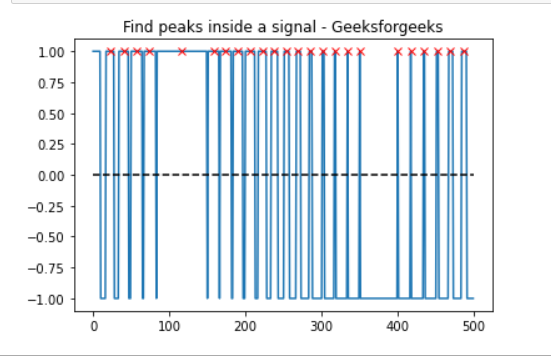Open in App
Not now

# Find all peaks amplitude lies above 0 Using Scipy

• Last Updated : 06 Jul, 2022

Prerequisites: Matplotlib, Scipy

In this article, we will see how to find all ‘x’ point above 0 with the help of find_peaks( ) function, that takes a 1-D array and finds all local maxima by a simple comparison of neighboring values.

Approach:

• Import required module.
• Find peaks inside a signal based on find_peaks() properties.
• Label the graph.
• Display Graph.

Step 1: Import all libraries.

## Python3

 `import` `matplotlib.pyplot as plt``import` `numpy as np``from` `scipy.signal ``import` `find_peaks``from` `scipy ``import` `signal`

Step 2: electrocardiogram(): The returned signal is a 5-minute-long electrocardiogram (ECG), a medical recording of the heart’s electrical activity, sampled at 360 Hz.

Syntax:

scipy.signal.find_peaks(x, height=None)

Parameter:

• x: A signal with peaks.
• height:  Required height of peaks. Either a number, None,

Return:

peaks: Indices of peaks in x that satisfy all given conditions.
peak_heights:  If the height is given, the height of each peak is x.

## Python3

 `import` `matplotlib.pyplot as plt``import` `numpy as np``from` `scipy.signal ``import` `find_peaks``from` `scipy ``import` `signal` `t ``=` `np.linspace(``0``, ``1``, ``500``, endpoint``=``False``)``sig ``=` `np.sin(``2` `*` `np.pi ``*` `t)``x``=` `signal.square(``2` `*` `np.pi ``*` `30` `*` `t, duty``=``(sig ``+` `1``)``/``2``)``peak, _ ``=` `find_peaks(x, height``=``0``)`

Below is the full Implementation:

## Python3

 `import` `matplotlib.pyplot as plt``import` `numpy as np``from` `scipy.signal ``import` `find_peaks``from` `scipy ``import` `signal` `t ``=` `np.linspace(``0``, ``1``, ``500``, endpoint``=``False``)``sig ``=` `np.sin(``2` `*` `np.pi ``*` `t)``x``=` `signal.square(``2` `*` `np.pi ``*` `30` `*` `t, duty``=``(sig ``+` `1``)``/``2``)``peak, _ ``=` `find_peaks(x, height``=``0``)` `plt.plot(x)``plt.title(``"Find peaks inside a signal - Geeksforgeeks"``)``plt.plot(peak, x[peak], ``"x"``, color ``=` `'r'``)``plt.plot(np.zeros_like(x), ``"--"``, color``=``"black"``)``plt.show()`

Output:My Personal Notes arrow_drop_up